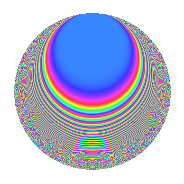# Properties

 Label 731.2.d.dLevel 731 Weight 2 Character orbit 731.d Analytic conductor 5.837 Analytic rank 0 Dimension 34 CM No

# Related objects

## Newspace parameters

 Level: $$N$$ = $$731 = 17 \cdot 43$$ Weight: $$k$$ = $$2$$ Character orbit: $$[\chi]$$ = 731.d (of order $$2$$ and degree $$1$$)

## Newform invariants

 Self dual: No Analytic conductor: $$5.83706438776$$ Analytic rank: $$0$$ Dimension: $$34$$ Sato-Tate group: $\mathrm{SU}(2)[C_{2}]$

## $q$-expansion

The dimension is sufficiently large that we do not compute an algebraic $$q$$-expansion, but we have computed the trace expansion.

 $$\operatorname{Tr}(f)(q) =$$ $$34q - 6q^{2} + 34q^{4} - 18q^{8} - 48q^{9} + O(q^{10})$$ $$\operatorname{Tr}(f)(q) =$$ $$34q - 6q^{2} + 34q^{4} - 18q^{8} - 48q^{9} + 16q^{13} - 8q^{15} + 26q^{16} + 14q^{18} + 16q^{19} + 20q^{21} - 44q^{25} - 26q^{26} + 88q^{30} - 42q^{32} + 12q^{33} - 42q^{34} + 22q^{35} + 34q^{38} - 14q^{42} - 34q^{43} + 30q^{47} - 62q^{49} - 46q^{50} - 10q^{51} + 26q^{52} - 46q^{53} + 16q^{55} - 20q^{59} - 42q^{60} + 102q^{64} - 70q^{66} - 32q^{67} - 10q^{68} + 74q^{69} + 130q^{70} + 22q^{72} + 38q^{76} - 78q^{77} + 46q^{81} - 60q^{83} - 98q^{84} - 38q^{85} + 6q^{86} + 16q^{87} + 26q^{89} + 14q^{93} - 78q^{94} + 78q^{98} + O(q^{100})$$

## Embeddings

For each embedding $$\iota_m$$ of the coefficient field, the values $$\iota_m(a_n)$$ are shown below.

For more information on an embedded modular form you can click on its label.

Label $$a_{2}$$ $$a_{3}$$ $$a_{4}$$ $$a_{5}$$ $$a_{6}$$ $$a_{7}$$ $$a_{8}$$ $$a_{9}$$ $$a_{10}$$
560.1 −2.79374 1.46205i 5.80499 1.65611i 4.08460i 2.54474i −10.6302 0.862404 4.62675i
560.2 −2.79374 1.46205i 5.80499 1.65611i 4.08460i 2.54474i −10.6302 0.862404 4.62675i
560.3 −2.48305 0.676403i 4.16556 3.88874i 1.67955i 2.90905i −5.37720 2.54248 9.65595i
560.4 −2.48305 0.676403i 4.16556 3.88874i 1.67955i 2.90905i −5.37720 2.54248 9.65595i
560.5 −2.34774 2.97035i 3.51191 3.06850i 6.97362i 3.33516i −3.54957 −5.82298 7.20405i
560.6 −2.34774 2.97035i 3.51191 3.06850i 6.97362i 3.33516i −3.54957 −5.82298 7.20405i
560.7 −1.90092 2.97496i 1.61349 1.24660i 5.65517i 4.11114i 0.734723 −5.85042 2.36968i
560.8 −1.90092 2.97496i 1.61349 1.24660i 5.65517i 4.11114i 0.734723 −5.85042 2.36968i
560.9 −1.52896 1.41141i 0.337716 1.28480i 2.15799i 2.51989i 2.54156 1.00791 1.96441i
560.10 −1.52896 1.41141i 0.337716 1.28480i 2.15799i 2.51989i 2.54156 1.00791 1.96441i
560.11 −1.28013 2.62270i −0.361257 1.51509i 3.35741i 3.46119i 3.02273 −3.87855 1.93952i
560.12 −1.28013 2.62270i −0.361257 1.51509i 3.35741i 3.46119i 3.02273 −3.87855 1.93952i
560.13 −1.27937 0.638162i −0.363207 1.64459i 0.816447i 4.36496i 3.02342 2.59275 2.10404i
560.14 −1.27937 0.638162i −0.363207 1.64459i 0.816447i 4.36496i 3.02342 2.59275 2.10404i
560.15 −0.811392 2.87350i −1.34164 2.95301i 2.33154i 2.64644i 2.71138 −5.25702 2.39605i
560.16 −0.811392 2.87350i −1.34164 2.95301i 2.33154i 2.64644i 2.71138 −5.25702 2.39605i
560.17 −0.335917 0.523814i −1.88716 1.51365i 0.175958i 0.357736i 1.30576 2.72562 0.508460i
560.18 −0.335917 0.523814i −1.88716 1.51365i 0.175958i 0.357736i 1.30576 2.72562 0.508460i
560.19 0.366222 1.80029i −1.86588 2.01471i 0.659307i 2.54407i −1.41577 −0.241043 0.737831i
560.20 0.366222 1.80029i −1.86588 2.01471i 0.659307i 2.54407i −1.41577 −0.241043 0.737831i
See all 34 embeddings
 $$n$$: e.g. 2-40 or 990-1000 Embeddings: e.g. 1-3 or 560.34 Significant digits: Format: Complex embeddings Normalized embeddings Satake parameters Satake angles

## Inner twists

This newform does not have CM; other inner twists have not been computed.

## Hecke kernels

This newform can be constructed as the kernel of the linear operator $$T_{2}^{17} + \cdots$$ acting on $$S_{2}^{\mathrm{new}}(731, [\chi])$$.